2019-01-20 10:30:10 weixin_43786907 阅读数 885
• ###### LCD1602和12864显示器-第1季第17部分

本课程是《朱有鹏老师单片机完全学习系列课程》第1季第17个课程，主要讲解LCD1602和LCD12864这两种单片机常用LCD显示器的显示原理、以及英文、中文、图片等如何编程显示。本课程对于显示编程具有重要的启蒙作用，是学习各种深入复杂的显示知识的基础。

4093 人正在学习 去看看 朱有鹏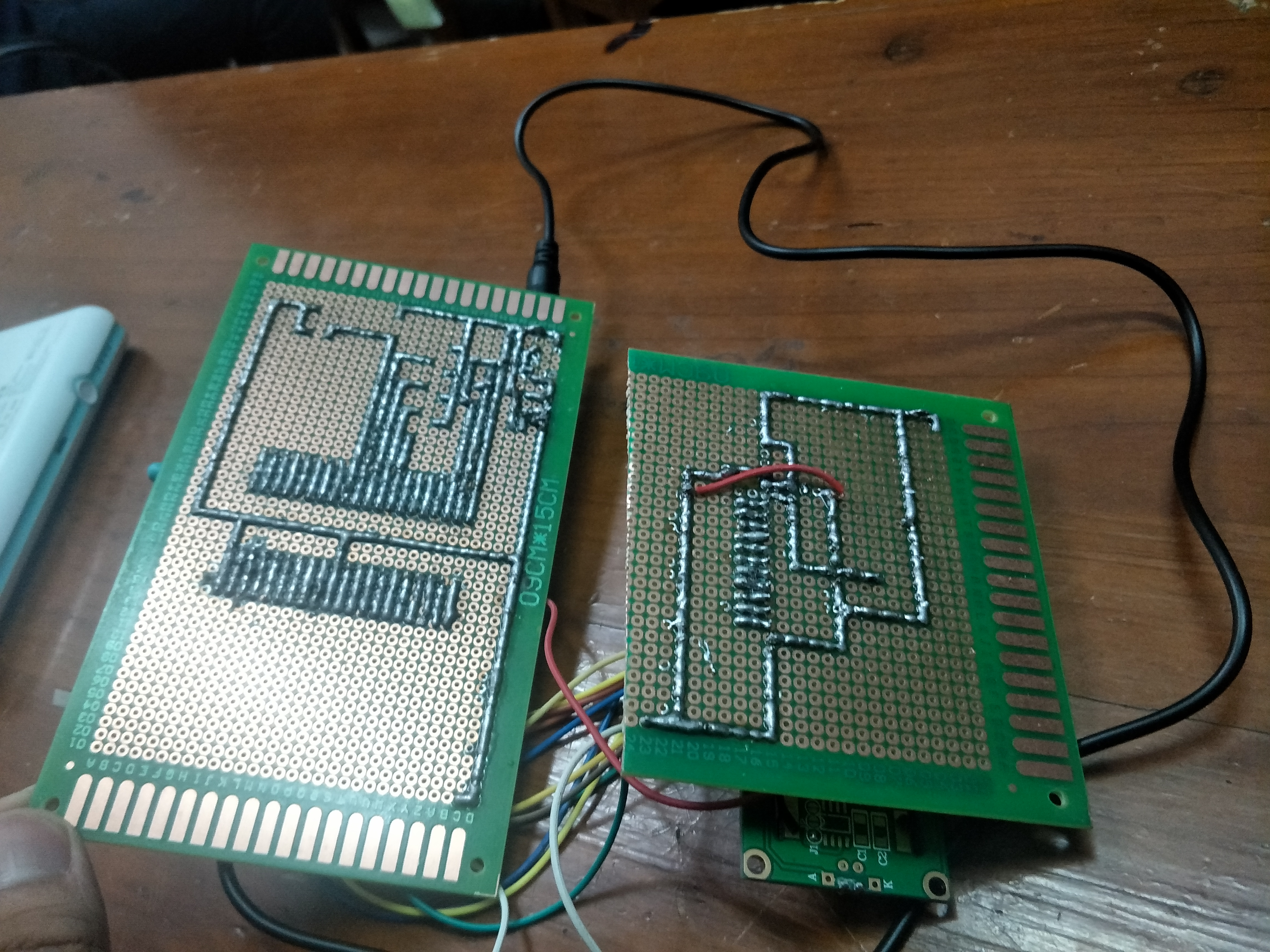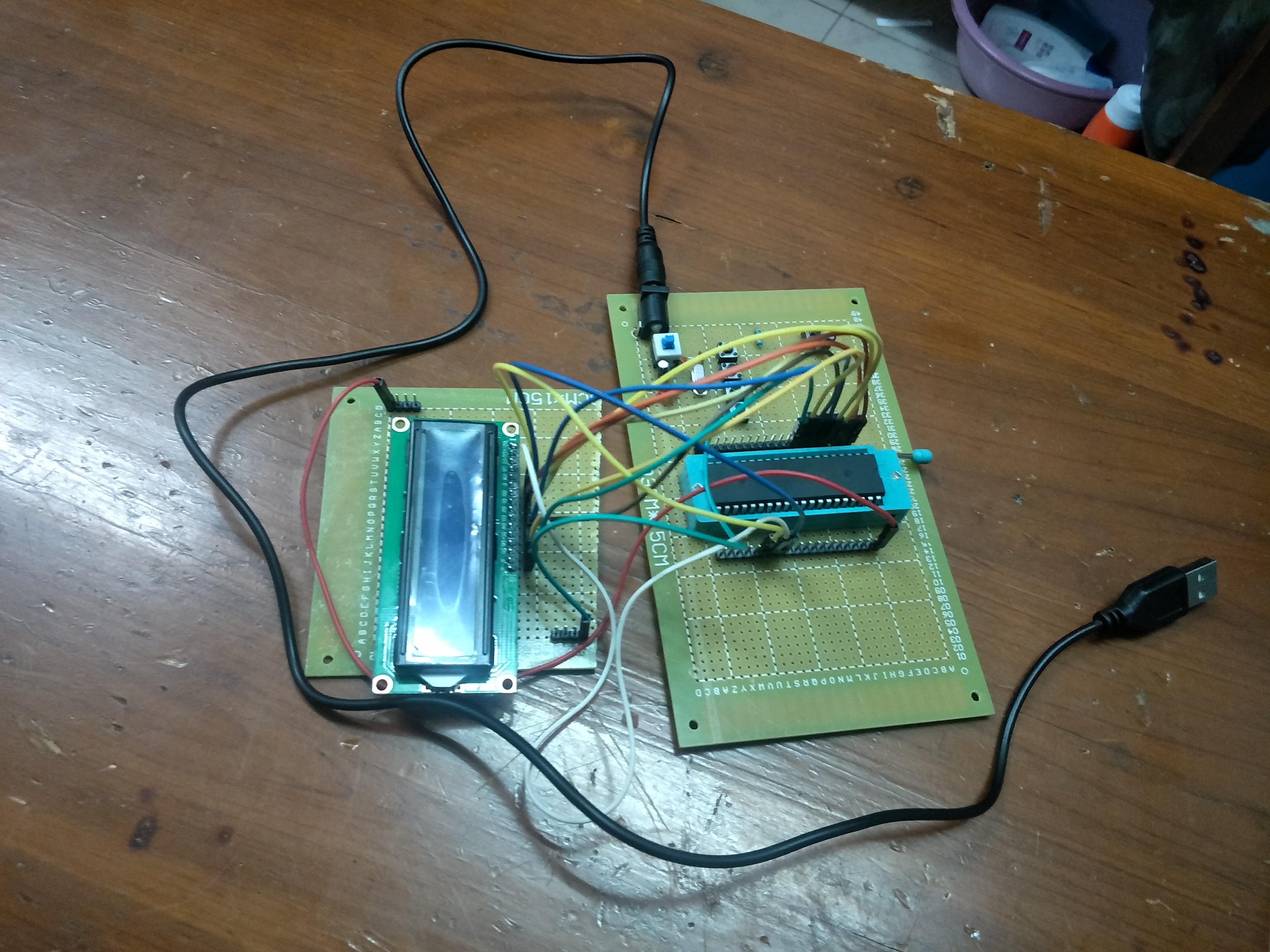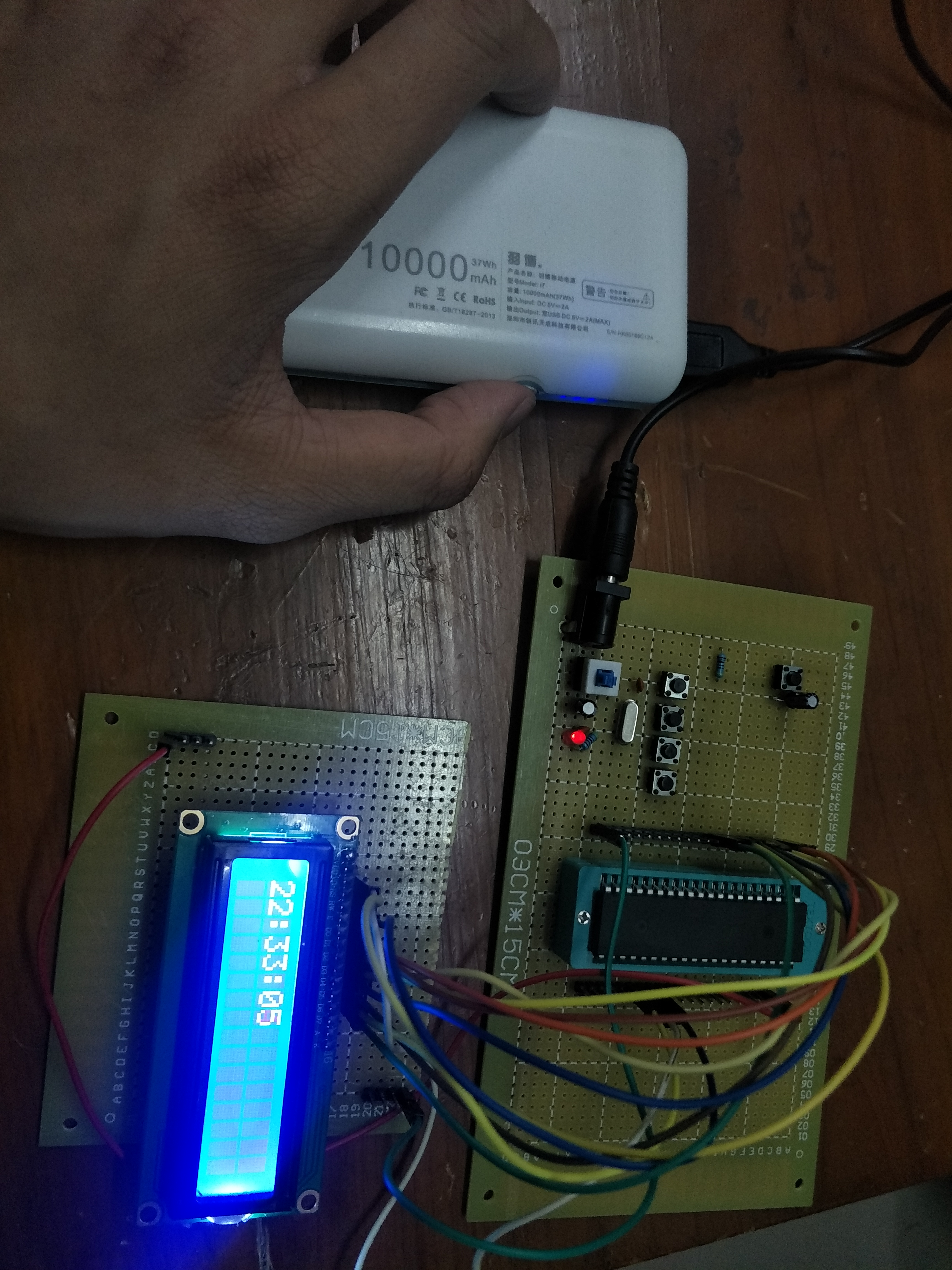2019-01-05 18:59:22 weixin_44414234 阅读数 2449
• ###### LCD1602和12864显示器-第1季第17部分

本课程是《朱有鹏老师单片机完全学习系列课程》第1季第17个课程，主要讲解LCD1602和LCD12864这两种单片机常用LCD显示器的显示原理、以及英文、中文、图片等如何编程显示。本课程对于显示编程具有重要的启蒙作用，是学习各种深入复杂的显示知识的基础。

4093 人正在学习 去看看 朱有鹏

# 51单片机LCD1602电子时钟

## 三.从中学到了什么

1.新部分：首先我了解到了lcd1602的工作方式，其中：Vss接电源地，Vcc接5V电源，RS与WR为输入令与数据端口，D0~D7为输入数据口。以及可以选择地址进行输入。
2.单片机部分：进一步了解了各种外部中断的的应用，如：在考虑四种模式和按键的关系上，考虑相互的优先级和需求，进一步规划
3.同时要灵活应用已有的各种条件，如在整点报时上要求“不影响主程序的延时”首先计时器已经满足不影响主程序，并且每次运行都有一个比较固定的时间，只要设定好次数就可以做到

## 四.未来计划

1.争取了解多种解决问题的方法
2.尝试自己读懂每个原件的使用说明，做到深入了解每个原件
3.了解下载模块，在焊接上发挥
4.深入了解c51单片机的性能（此次存在内存超出的情况）

1.未合理分配内存，导致内存超出

2.循环的失误导致闪屏

3.变量数据溢出

## 六.对本次要求的详细解读

1.首先，时钟显示模式需求的本质上还是数字的进位和显示的不断更新。再就是整点报时，这个可以通过位变化检测或者单纯的数字变化来确定蜂鸣器启动的时间点。
2.时钟调整模式要求对时分进行键位调整，当然，之后的时钟显示也应该是调整后的，可以采取先改变再对调，或者先暂停再改变。
3.再就是闹钟模式，闹钟模式调整上其实和调整模式差不多，关键就是设置一个值和时钟显示模式对应，让其能够再

4.倒计时其实关键并不在于显示和计时，因为这两点参考前面就行了，主要是可控性，也就是设置开关。

## 七.本次对自己个人觉得要注意的点

1.下面来满足这些需求，首先这里有四种模式，如果用两个外部中断肯定不够，况且0号口外度中断优先于计时器，

2. 显示里面需要注意的是每次最好要重新标志好初始的输入点，避免输入错位

## 八.有关错误的查找

``````#include <reg51.h>
#include <math.h>

sbit RS=P2^4;
sbit RW=P2^5;
sbit E=P2^6;
sbit BUZ=P1^0;
sbit IN=P2^0;
sbit DE=P2^1;
sbit BA=P2^2;

unsigned char code mod1[]=" workmod1:clock ";
unsigned char code mod2[]=" workmod2:setup ";
unsigned char code mod3[]=" workmod3:alarm ";
unsigned char code mod4[]=" workmod4:timer ";

unsigned int o,p;
unsigned char bs,i;
unsigned int c1,c2,c3,c4,c5,c6,z,i3;//mod1
unsigned int s;//mod2
long int a;
long int kk,k2;//mod4
long int t,k,i1,i2;//timer

void delay(int t)//50us
{
unsigned int x;
for(;t>0;t--)
for(x=19;x>0;x--);
}

void writecom(unsigned char com)
{
E=0;
RS=0;
RW=0;
P0=com;
delay(10);
E=1;
delay(20);
E=0;
}

void writedate(unsigned char dat)
{
E=0;
RS=1;
RW=0;
P0=dat;
delay(10);
E=1;
delay(20);
E=0;
}

void innit()
{
delay(30);
writecom(0x38);
delay(10);
writecom(0x38);
delay(10);
writecom(0x38);
writecom(0x38);
writecom(0x08);
writecom(0x01);
writecom(0x06);
writecom(0x0c);
}

void workmod1()
{
innit();
s=0;
while(bs==0)
{
writecom(0x80);
for(i=0;i<16;i++)
{
writedate(mod1[i]);
}
writecom(0x80+0x40);
if(s==0)writedate('O'),writedate('F'),writedate('F');
if(s==1)writedate('O'),writedate('N'),writedate(' ');
c1=i1%10,c2=i1/10,c3=i2%60%10,c4=i2%60/10,c5=i2/60%24%10,c6=i2/60%24/10;
writecom(0x80+0x45);
writedate(':');
writedate(':');
i3=i2/60;
if(i3!=z)//strike
{
o=1;
z=i3;
}
if(s==1&&a==i2)o=1;//alarm
}
}

void workmod2()
{
innit();
s=0;
writecom(0x80);
for(i=0;i<16;i++)
{
writedate(mod2[i]);
}
while(bs==1)
{
i1=0;
writecom(0x80+0x4a);
writedate(':');writedate('0');writedate('0');
writecom(0x80+0x40);
switch(s)
{
case 0:
{
writedate('M');
if(IN==0)
{
delay(500);
i2++;
delay(500);
}
else if(DE==0)
{
delay(500);
i2--;
delay(500);
}
}break;
case 1:
{
writedate('H');
if(IN==0)
{
delay(500);
i2=i2+60;
delay(500);
}
else if(DE==0)
{
delay(500);
i2=i2-60;
delay(500);
}
}break;
}
c1=i1%10,c2=i1/10,c3=i2%60%10,c4=i2%60/10,c5=i2/60%24%10,c6=i2/60%24/10;
writecom(0x80+0x45);
writedate(':');
}
}

void workmod3()
{
innit();
s=0;
writecom(0x80);
for(i=0;i<16;i++)
{
writedate(mod3[i]);
}
while(bs==2)
{
if(a==1440)a=0;
writecom(0x80+0x40);
switch(s)
{
case 0:
{
writedate('M');
if(IN==0)
{
delay(500);
a++;
delay(500);
}
else if(DE==0)
{
delay(500);
a--;
delay(500);
}
}break;
case 1:
{
writedate('H');
if(IN==0)
{
delay(500);
a=a+60;
delay(500);
}
else if(DE==0)
{
delay(500);
a=a+60;
delay(500);
}
}break;
}
writecom(0x80+0x45);
c3=a%60%10,c4=a%60/10,c5=a/60%10,c6=a/60/10;
writedate(':');
}
}

void workmod4()
{
innit();
kk=0;
writecom(0x80);
for(i=0;i<16;i++)
{
writedate(mod4[i]);
}
while(bs==3)
{
k2=0;
if(s==0)
{
writecom(0x80+0x45);
writedate('R');
writedate('E');
writedate('A');
writedate('D');
writedate('Y');
}
else if(s==1)
{
writecom(0x80+0x45);
for(i=0;i<5;i++)
writedate(' ');
while(kk!=60&&bs==3&&s==1)
{
kk=k2/20;
writecom(0x80+0x47);
}
if(kk==60)o=1,s=0,kk=0;
}
}
}

void main()
{
innit();
TMOD=0x01;TH0=(65536-50000)/256;TL0=(65536-50000)%256;
EA=1;EX0=1;EX1=1;
IT0=1;IT1=1;
ET0=1;TR0=1;
k=0;bs=0;s=0;z=0;k2=0;i1=0;i2=0;kk=0;a=0;o=0;p=0;
while(1)
{
switch(bs)
{
case 0:workmod1();break;
case 1:workmod2();break;
case 2:workmod3();break;
case 3:workmod4();break;
}
}
}

void int0 () interrupt 0//buttion:affirm
{
s++;s=s%2;
BUZ=1;
}

void timer0 () interrupt 1
{
for(t=0;t<20;t++)
{
TH0=(65536-50000)/256;
TL0=(65536-50000)%256;
}
k++;
if(k==20) i1++,k=0;
if(i1==60) i2++,i1=0;
if(i2==1440) i2=0;
k2++;//only mod4
if(o)BUZ=0,p++;
if(p==40)BUZ=1,o=0,p=0;
}

void int2 () interrupt 2//buttion:set up
{
kk=0;BUZ=1;
bs++;
bs=bs%4;
}
``````

2018-11-13 19:11:26 qq_43234586 阅读数 553
• ###### LCD1602和12864显示器-第1季第17部分

本课程是《朱有鹏老师单片机完全学习系列课程》第1季第17个课程，主要讲解LCD1602和LCD12864这两种单片机常用LCD显示器的显示原理、以及英文、中文、图片等如何编程显示。本课程对于显示编程具有重要的启蒙作用，是学习各种深入复杂的显示知识的基础。

4093 人正在学习 去看看 朱有鹏

## 单片机lcd1602分秒计时’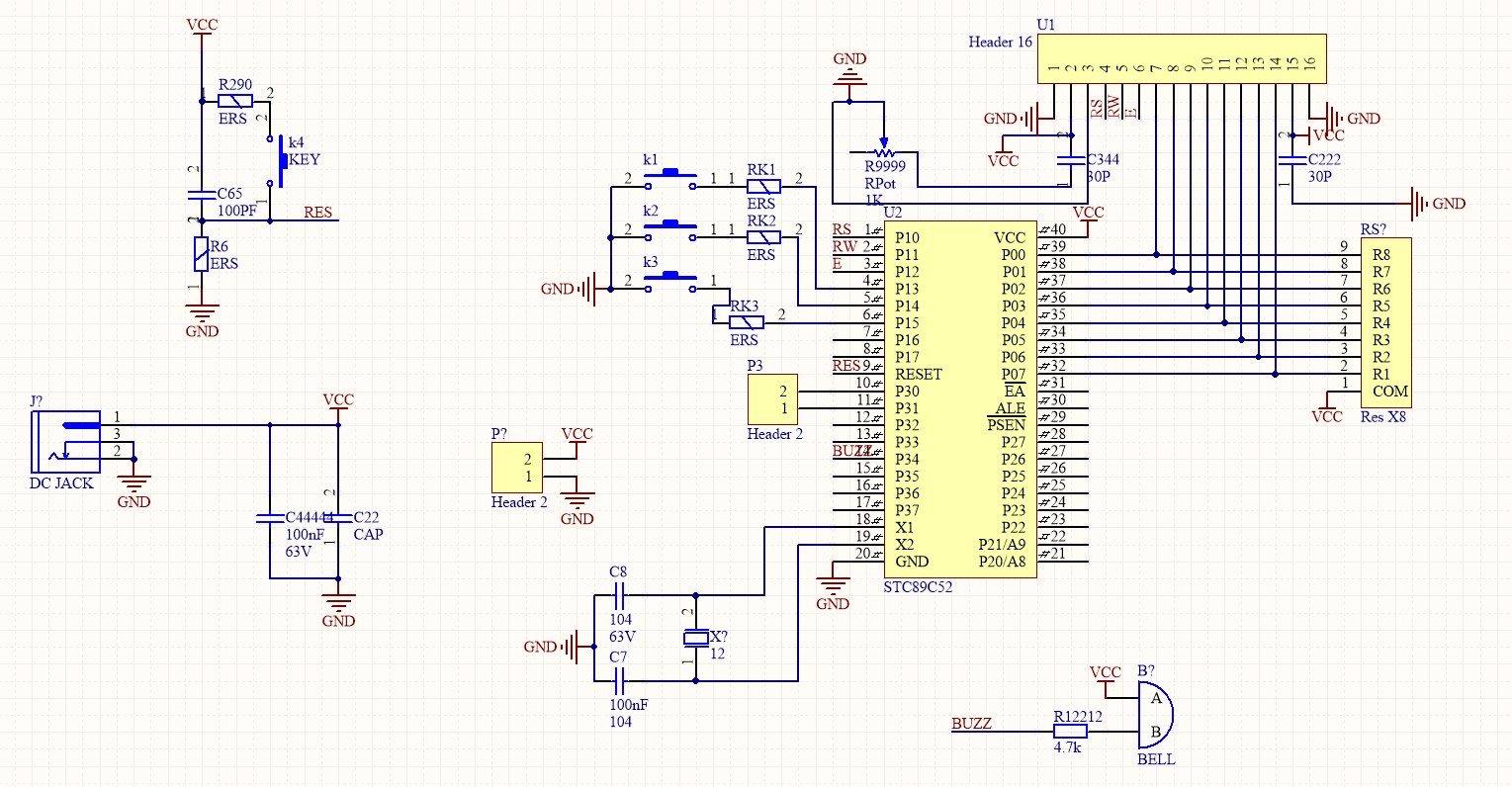pcb板尽量不使用跳线和多面线.

#include  <reg52.h>
#define uint unsigned int
#define uchar unsigned char
sbit rs=P1^0;
sbit rw=P1^1;
sbit e=P1^2;
sbit beep=P3^4;
sbit key0=P3^1;
sbit key1=P3^0;
sbit key2=P3^2;
sbit key3=P3^3;
uchar table1[]=“012345”;//////////////¶¨ÒåÊý×é/////////////
uchar table2[]=“0123456789”;/////////¶¨ÒåÊý×é///////
uchar table3[]=":";/////////////////¶¨ÒåÊý×é///////
uchar i,j,m,q,num=0,u=0,flag=0;/////////////¶¨Òå//////////////////
void delay_50ms(uint t)            //50msÑÓÊ±
{
uint j;
for(;t>0;t–)
for(j=6245;j>0;j–);
}
void delay_50us(uint t)            //50usÑÓÊ±
{
uchar j;
for(;t>0;t–)
for(j=19;j>0;j–);
}
void write_com(uchar com)///////////ÕÒlcd1602Î»ÖÃ/////
{
e=0;
rs=0;
rw=0;
P0=com;
delay_50us(10);
e=1;
delay_50us(20);
e=0;
}
void write_data(uchar dat)    ///////////////////////////////////////
{
e=0;
rs=1;
rw=0;
P0=dat;
delay_50us(10);
e=1;
delay_50us(20);
e=0;
}
void init(void)
{
delay_50us(300);
write_com(0x38);
delay_50us(100);
write_com(0x38);
delay_50us(100);
write_com(0x38);
write_com(0x38);
write_com(0x08);
write_com(0x01);
write_com(0x06);
write_com(0x0c);
}
void write_0()////////////////³õÊ¼»¯00:00:00//////////////////
{
write_data(table1);
write_data(table2);
write_data(table3);
write_data(table1);
write_data(table2);
write_data(table3);
write_data(table1);
write_data(table2);
}
void write_1()/////////////////³õÊ¼»¯00:01:00//////////////µ¹¼ÆÊ±//////////
{
write_data(table1);
write_data(table2);
write_data(table3);
write_data(table1);
write_data(table2);
write_data(table3);
write_data(table1);
write_data(table2);
}
void shijian(int q,w,e,r,t,y,num,c,sum)///////////////////////////Ê±¼ä//////////////////
{
TMOD=0x01;
TH0=(65536-50000)/256;
TL0=(65536-50000)%256;
EA=1;
ET0=1;
TR0=1;
num=0;sum=0;
for(y=0;y<10;y++)////////////////////////////////0x80/////
{
write_com(0x80);write_data(table2[y]);
for(t=0;t<10;t++)/////////////////////////////0x80+0x01
{
write_com(0x80+0x01);write_data(table1[t]);
while(sum1)
{
u=0;
if(sum
0)
break;
if(u0)
{
while(1)
{
beep=~beep;
delay_50us(10);
if(u
2)
{
beep=0;
sum=0;
break;
}
}
}
}
sum=1;
for(r=0;r<6;r++)///////////////////////////0x80+0x03
{
write_com(0x80+0x03);write_data(table1[r]);
for(e=0;e<10;e++)////////////////////////0x80/+0x04
{
write_com(0x80+0x04);write_data(table2[e]);
while(num1)////////////////////fmqi·äÃùÆ÷//////
{
u=0;
if(num
0)
break;
if(u0)
{
while(1)
{
beep=~beep;
delay_50us(10);
if(u
2)
{
beep=0;
num=0;
break;
}
}
}
}
num=1;/////////////·äÃùÆ÷//////////////////////////////////////////
for(w=0;w<6;w++)/////////////////////////0x80+0x06
{
write_com(0x80+0x06);write_data(table1[w]);
for(q=0;q<10;q++)///////////////////////0x80+0x07
{
if(key10)
{
delay_50us(10);
if(key1
0)
w++;
if(w6)
{
w=0;
write_com(0x80+0x06);write_data(table1[w]);
e++;
if(e
10)
{
e=0;
write_com(0x80+0x04);write_data(table2[e]);
r++;
if(r6)
{
r=0;
write_com(0x80+0x03);write_data(table1[r]);
t++;
if(t
10)
{
t=0;
write_com(0x80+0x01);write_data(table2[t]);
y++;
write_com(0x80);write_data(table2[y]);
}
}
}
}
write_com(0x80+0x06);write_data(table1[w]);
write_com(0x80+0x04);write_data(table2[e]);
write_com(0x80+0x03);write_data(table1[r]);
write_com(0x80+0x01);write_data(table2[t]);
write_com(0x80);write_data(table2[y]);
delay_50us(10);
while(!key1);
}
if(key20)
{
delay_50us(10);
if(key2
0)
e++;
if(e10)
{
e=0;
write_com(0x80+0x04);
write_data(table2[e]);
r++;
if(r
6)
{
r=0;
write_com(0x80+0x03);write_data(table1[r]);
t++;
if(t10)
{
t=0;
write_com(0x80+0x01);write_data(table2[t]);
}
}
}
write_com(0x80+0x04);write_data(table2[e]);
write_com(0x80+0x03);write_data(table1[r]);
write_com(0x80+0x01);write_data(table2[t]);
write_com(0x80);write_data(table2[y]);
while(!key2);
}
write_com(0x80+0x07);write_data(table2[q]);
u=0;
if(u
20)u=0;
if(key30)
{
flag=1;break;
}
}if(flag
1)break;
}if(flag1)break;
}if(flag
1)break;
}if(flag1)break;
}if(flag
1)break;
}
}
void daojishi(int s,t)/////////////////////µ¹¼ÆÊ±¿ªÊ¼///////////////
{
delay_50ms(20);
write_com(0x80+0x04);
write_data(table1);
for(s=5;s>-1;s–)
{
write_com(0x80+0x06);
write_data(table1[s]);
for(t=9;t>-1;t–)
{
write_com(0x80+0x07);
write_data(table2[t]);
delay_50ms(20);
}
}
}//////////////////////////////////////////////////////////////////////////////////////////
void main()
{
if(key00)
{
while(1)
{
if(key3
0)
{
init();
write_com(0x80);
write_1();
daojishi();
while(1);
}
init();
write_com(0x80);
write_0();
shijian();
}
}
}
void time0 () interrupt 1
{
TH0=(65536-50000)/256;
TL0=(65536-50000)%256;
u++;
}

2019-05-18 16:12:31 imxlw00 阅读数 112
• ###### LCD1602和12864显示器-第1季第17部分

本课程是《朱有鹏老师单片机完全学习系列课程》第1季第17个课程，主要讲解LCD1602和LCD12864这两种单片机常用LCD显示器的显示原理、以及英文、中文、图片等如何编程显示。本课程对于显示编程具有重要的启蒙作用，是学习各种深入复杂的显示知识的基础。

4093 人正在学习 去看看 朱有鹏

LCD1602为工业字符型液晶，LCD表示LiquidCrystal Display。能够同时显示16 x 2（16列2行）即32个字符。1602液晶也叫1602字符型液晶，它是一种专门用来显示字母、数字、符号等的点阵型液晶模块。它由若干个5X7或者5X11等点阵字符位组成，每个点阵字符位都可以显示一个字符，每位之间有一个点距的间隔，每行之间也有间隔，起到了字符间距和行间距的作用，正因为如此所以它不能很好地显示图形。

### 16条引脚定义

1 VSS 电源地 9 D2 数据端口
2 VDD 电源正极 10 D3 数据端口
3 VL 偏压信号 11 D4 数据端口
4 RS 命令/数据 12 D5 数据端口
5 R/W 读/写 13 D6 数据端口
6 E 使能 14 D7 数据端口
7 D0 数据端口 15 A 背光正极
8 D1 数据端口 16 K 背光负极

1. VSS接电源地。

2. VDD接+5V。

3. VO是液晶显示的偏压信号，可接10K的3296精密电位器。或同样阻值的RM065/RM063蓝白可调电阻。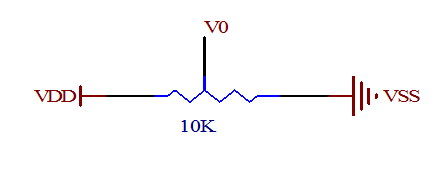4. RS是命令/数据选择引脚，接单片机的一个I/O，当RS为低电平时，选择命令；当RS为高电平时，选择数据。

5. RW是读/写选择引脚，接单片机的一个I/O，当RW为低电平时，向LCD1602写入命令或数据；当RW为高电平时，从LCD1602读取状态或数据。如果不需要进行读取操作，可以直接将其接VSS。

6. E，执行命令的使能引脚，接单片机的一个I/O。

7. D0—D7，并行数据输入/输出引脚，可接单片机的P0—P3任意的8个I/O口。如果接P0口，P0口应该接4.7K—10K的上拉电阻。如果是4线并行驱动，只须接4个I/O口。

8. A背光正极，可接一个10—47欧的限流电阻到VDD。

9. K背光负极，接VSS。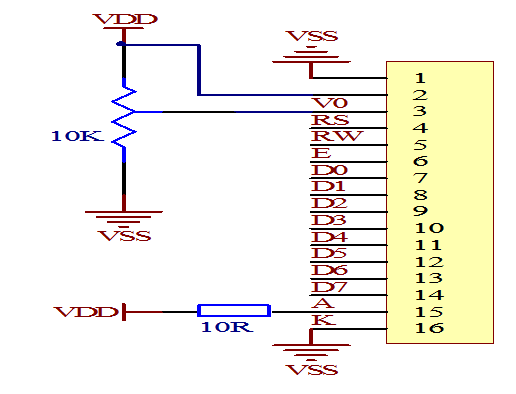### 基本操作

LCD1602的基本操作分为四种：

1. 读状态：输入RS=0，RW=1，E=高脉冲。输出：D0—D7为状态字。

2. 读数据：输入RS=1，RW=1，E=高脉冲。输出：D0—D7为数据。

3. 写命令：输入RS=0，RW=0，E=高脉冲。输出：无。

4. 写数据：输入RS=1，RW=0，E=高脉冲。输出：无。

• 读操作时序图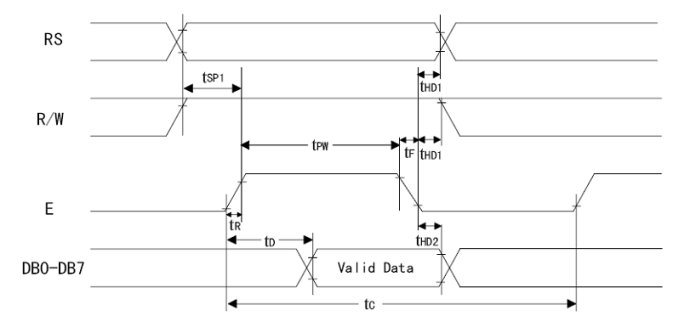• 写操作时序图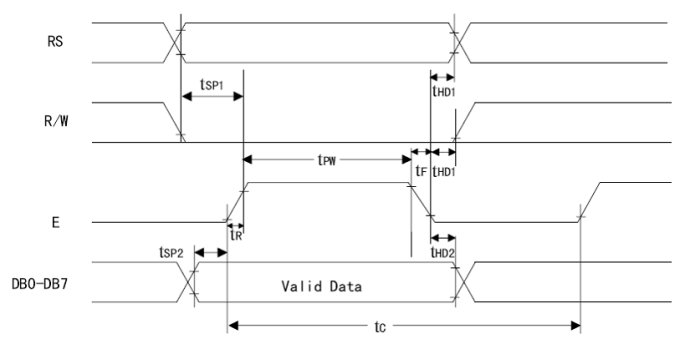• 时序时间参数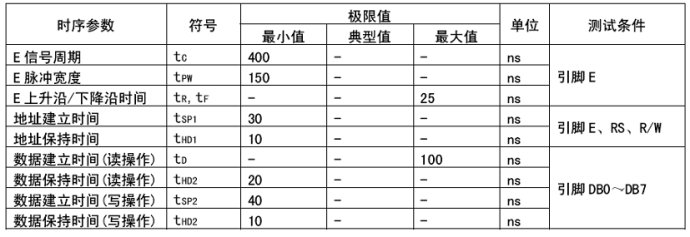### 工作方式设置指令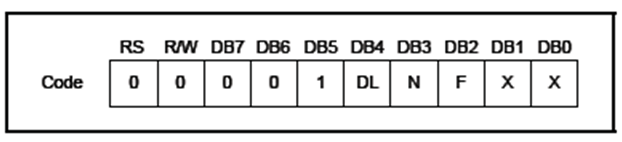×：不关心，也就是说这个位是0或1都可以，一般取0。

DL：设置数据接口位数。

DL=1：8位数据接口(D7—D0)。

DL=0：4位数据接口(D7—D4)。

N=0：一行显示。

N=1：两行显示。

F=0：5×8点阵字符。

F=1：5×10点阵字符。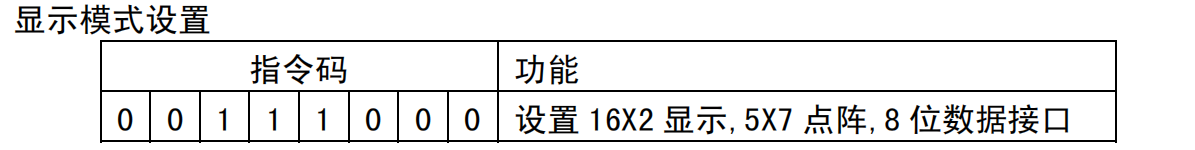### 显示开关控制指令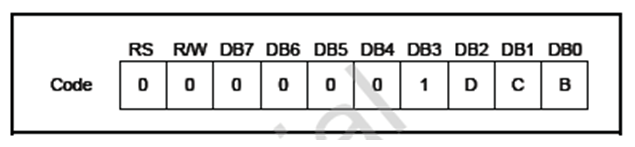D=1：显示开，D=0：显示关。

C=1：光标显示，C=0：光标不显示。

B=1：光标闪烁，B=0：光标不闪烁。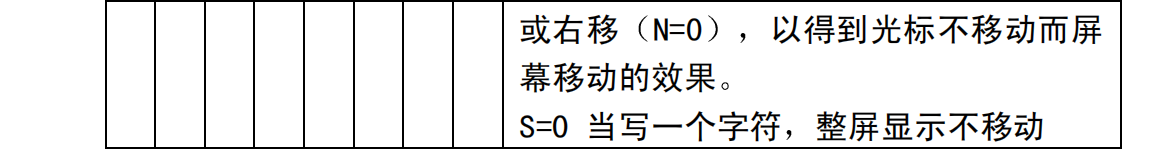### 数据指针设置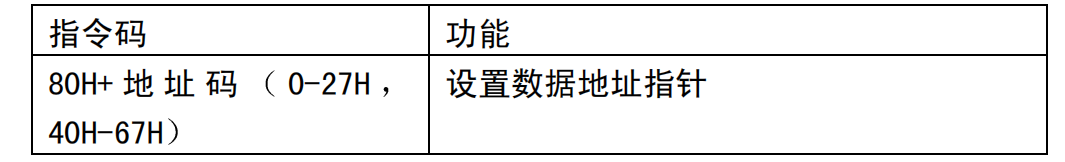### 其它设置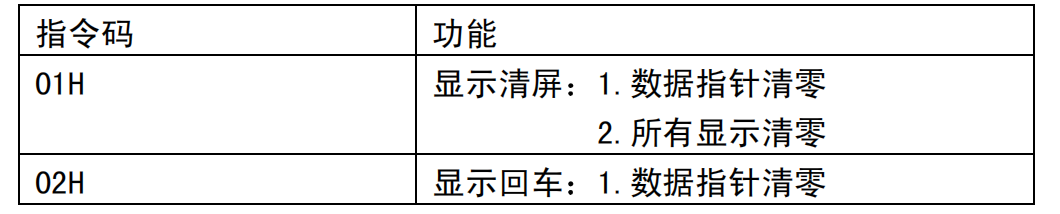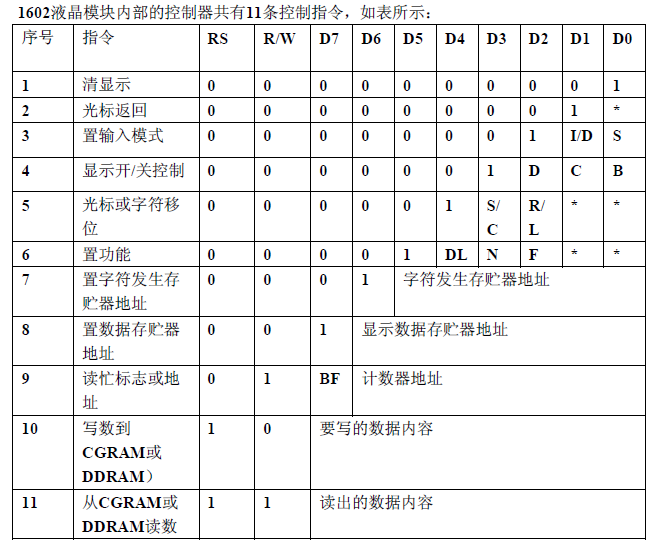### 编程思路

*设置1602液晶的功能；

*设置1602液晶的输入方式；

*设置1602液晶的显示方式；

*清屏；

2019-03-13 20:56:09 qq_39768922 阅读数 200
• ###### LCD1602和12864显示器-第1季第17部分

本课程是《朱有鹏老师单片机完全学习系列课程》第1季第17个课程，主要讲解LCD1602和LCD12864这两种单片机常用LCD显示器的显示原理、以及英文、中文、图片等如何编程显示。本课程对于显示编程具有重要的启蒙作用，是学习各种深入复杂的显示知识的基础。

4093 人正在学习 去看看 朱有鹏

/单片机lcd1602液晶显示/
#include"reg52.h"
#define u8 unsigned char //定义变量数据类型
#define u16 unsigned int
sbit rs=P2^6;//写数据/命令Io口
sbit wr=P2^5;//读/写操作IO口
sbit lcd_en=P2^7;//lcd使能端
u16 i,j;
u8 num;
u8 code table1[]=“If you want”;//用数组存放字符串（别漏掉双引号）
u8 code table2[]=“Just do it!!”;//若是单个字符则用单引号
void delay(u16);//ms级延时函数声明
void write_com(u8 com);//lcd写命令函数声明
void write_data(u8);//lcd写数据函数声明
void lcd_init();//lcd初始化函数声明
void main()//主函数
{
lcd_init();//lcd初始化
for(num=0;num<11;num++)//有多少个字符，就循环几次
{
write_data(table1[num]);//依次显示数组1中的字符
delay(300);//延时
}
write_com(0X80+0X40);//0X80+0X40为第二行的数据地址，表示下面的字符串显示在第二行
for(num=0;num<12;num++)//有多少个字符，就循环几次
{
write_data(table2[num]);//依次显示数组1中的字符
delay(300);
}
while(1);//程序在这停止
}
void write_com(u8 com)//lcd写命令函数
{
rs=0;//数据命令选择端，写命令时为0
wr=0;//读/写选择端，写数据时为0
lcd_en=0;//低电平时写入数据
P0=com;//将com写进P0中
lcd_en=1;//关闭使能端
delay(5);//延时
lcd_en=0;
}
void write_data(u8 dat)//lcd写数据函数
{
rs=1;//数据命令选择端，写数据时为0
wr=0;//读/写选择端，写数据时为0
lcd_en=0;
P0=dat;//将要写的数据发送到数据总线上
lcd_en=1;
delay(5);
lcd_en=0;
}
void lcd_init()//lcd初始化函数
{
write_com(0X38);//显示模式设置，设置为16x2显示
write_com(0X08);//显示关闭
write_com(0X01);//显示清屏（即数据指针清零，所有显示清零）
write_com(0X06);//显示光标移动设置，即写一个字符后地址指针自动加1，且光标加1
write_com(0X0F);//显示开启和光标设置（即开显示，显示光标，把光标设置为闪烁）
}
void delay(u16 x)//ms延时函数
{
for(i=x;i>0;i–)
{
for(j=120;j>0;j–);
}
}